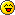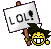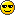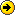# Three equations of motion- to derive graphically##Re: Three equations of motion- to derive graphically##Re: Three equations of motion- to derive graphically

The three equations of motion v = u + at ; s = ut + (1/2) at2 and v2 = u2 + 2as can be derived with the help of graphs as described below.
1. Derive v = u + at by Graphical Method
Consider the velocity – time graph of a body shown in the below Figure.Velocity–Time graph to derive the equations of motion.
The body has an initial velocity u at point A and then its velocity changes at a uniform rate from A to B in time t. In other words, there is a uniform acceleration 'a' from A to B, and after time t its final velocity becomes 'v' which is equal to BC in the graph. The time t is represented by OC. To complete the figure, we draw the perpendicular CB from point C, and draw AD parallel to OC. BE is the perpendicular from point B to OE.
Now, Initial velocity of the body, u = OA...... (1)
And, Final velocity of the body, v = BC........ (2)
But from the graph BC = BD + DC
Therefore, v = BD + DC ......... (3)
Again DC = OA
So, v = BD + OA
Now, From equation (1), OA = u
So, v = BD + u ........... (4)
We should find out the value of BD now. We know that the slope of a velocity – time graph is equal to acceleration, a.
Thus, Acceleration, a = slope of line AB
or a = BD/AD
But AD = OC = t,
so putting t in place of AD in the above relation, we get:
a = BD/t
or BD = at
Now, putting this value of BD in equation (4) we get :
v = at + u
This equation can be rearranged to give:
v = u + at
And this is the first equation of motion. It has been derived here by the graphical method.
2. Derive s = ut + (1/2) at2 by Graphical Method
Velocity–Time graph to derive the equations of motion.
Suppose the body travels a distance s in time t. In the above Figure, the distance travelled by the body is given by the area of the space between the velocity – time graph AB and the time axis OC,which is equal to the area of the figure OABC. Thus:
Distance travelled = Area of figure OABC
= Area of rectangle OADC + Area of triangle ABD
We will now find out the area of the rectangle OADC and the area of the triangle ABD.
(i) Area of rectangle OADC = OA × OC
= u × t
= ut ...... (5)
(ii) Area of triangle ABD = (1/2) × Area of rectangle AEBD
= (1/2) × AD × BD
= (1/2) × t × at (because AD = t and BD = at)
= (1/2) at2...... (6)
So, Distance travelled, s = Area of rectangle OADC + Area of triangle ABD
or s = ut + (1/2) at2
This is the second equation of motion. It has been derived here by the graphical method.
3. Derive v2 = u2 + 2as by Graphical Method
Velocity–Time graph to derive the equations of motion.
We have just seen that the distance travelled s by a body in time t is given by the area of the figure OABC which is a trapezium. In other words,
Distance travelled, s = Area of trapezium OABC

Now, OA + CB = u + v and OC = t. Putting these values in the above relation, we get:
...... (7)
We now want to eliminate t from the above equation. This can be done by obtaining the value of t from the first equation of motion. Thus, v = u + at (First equation of motion)
And, at = v – u or
Now, putting this value of t in equation (7) above, we get:
or 2as = v2 – u2 [because (v + u) × (v – u) = v2 – u2]
or v2 = u2 + 2as
This is the third equation of motion.

I hope u like it..

##Re: Three equations of motion- to derive graphically

NOW TRY THIS OUT!!!!Consider an object moving with a uniform velocity u in a straight line. Let it be given a uniform acceleration a at time t = 0 when its initial velocity is u. As a result of the acceleration, its velocity increases to v (final velocity) in time t and S is the distance covered by the object in time t.
The figure shows the velocity-time graph of the motion of the object.
Slope of the v - t graph gives the acceleration of the moving object.
Thus, acceleration = slope = AB =

v - u = at
v = u + at  I equation of motion

Graphical Derivation of Second Equation
Distance travelled S = area of the trapezium ABDO
= area of rectangle ACDO + area of DABC

(v = u + at I eqn of motion; v - u = at)

Graphical Derivation of Third Equation
S = area of the trapezium OABD.

Substituting the value of t in equation (1) we get,

2aS = (v + u) (v - u)
(v + u)(v - u) = 2aS [using the identity a2 - b2 = (a+b) (a-b)]
v2 - u2 = 2aS  III Equation of Motion

##Re: Three equations of motion- to derive graphically

Consider an object moving with a uniform velocity u in a straight line. Let it be given a uniform acceleration a at time t = 0 when its initial velocity is u. As a result of the acceleration, its velocity increases to v (final velocity) in time t and S is the distance covered by the object in time t.
The figure shows the velocity-time graph of the motion of the object.
Slope of the v - t graph gives the acceleration of the moving object.
Thus, acceleration = slope = AB =

v - u = at
v = u + at I equation of motion

Graphical Derivation of Second Equation
Distance travelled S = area of the trapezium ABDO
= area of rectangle ACDO + area of DABC

(v = u + at I eqn of motion; v - u = at)

Graphical Derivation of Third Equation
S = area of the trapezium OABD.

Substituting the value of t in equation (1) we get,

2aS = (v + u) (v - u)
(v + u)(v - u) = 2aS [using the identity a2 - b2 = (a+b) (a-b)]
v2 - u2 = 2aS III Equation of Motion

##Re: Three equations of motion- to derive graphically

WATCH THIS OUT !!!!##Re: Three equations of motion- to derive graphically

Hey Guys!!! Its interesting...Motion

Equation for Velocity – Time relation by graphical method – First equation of Motion –

Let an object is moving with uniform acceleration.

Velocity Time Graph
Let the initial velocity of the object = u

Let the object is moving with uniform acceleration, a.

Let object reaches at point B after time, t and its final velocity becomes, v

Draw a line parallel to x-axis DA from point, D from where object starts moving.

Draw another line BA from point B parallel to y-axis which meets at E at y-axis.

Let OE = time, t

Now, from the graph,

BE = AB + AE

⇒ v = DC + OD (Since, AB = DC and AE = OD)

⇒ v = DC + u (Since, OD = u)

⇒ v = DC + u ------------------- (i)

Class 9 Motion
Above equation is the relation among initial velocity (u), final velocity (u), acceleration (a) and time (t). It is called first equation of motion.##Three equations of motion- to derive graphically

how can the equations of motion b derived graphically?

##Re: Three equations of motion- to derive graphically